# Random Forest Regression in Python

Every decision tree has high variance, but when we combine all of them together in parallel then the resultant variance is low as each decision tree gets perfectly trained on that particular sample data and hence the output doesn’t depend on one decision tree but multiple decision trees. In the case of a classification problem, the final output is taken by using the majority voting classifier. In the case of a regression problem, the final output is the mean of all the outputs. This part is Aggregation.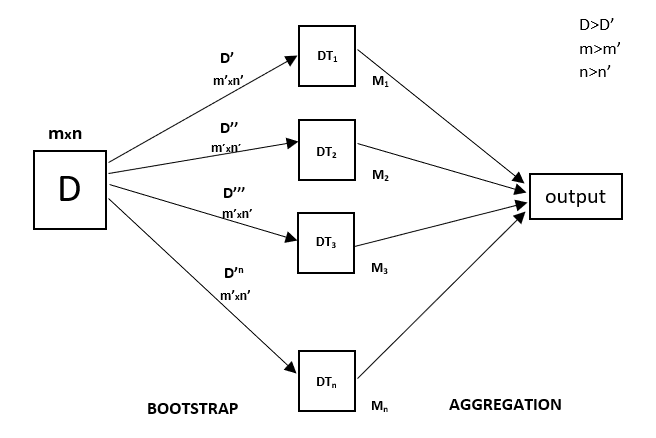A Random Forest is an ensemble technique capable of performing both regression and classification tasks with the use of multiple decision trees and a technique called Bootstrap and Aggregation, commonly known as bagging. The basic idea behind this is to combine multiple decision trees in determining the final output rather than relying on individual decision trees.
Random Forest has multiple decision trees as base learning models. We randomly perform row sampling and feature sampling from the dataset forming sample datasets for every model. This part is called Bootstrap.

We need to approach the Random Forest regression technique like any other machine learning technique

• Design a specific question or data and get the source to determine the required data.
• Make sure the data is in an accessible format else convert it to the required format.
• Specify all noticeable anomalies and missing data points that may be required to achieve the required data.
• Create a machine learning model
• Set the baseline model that you want to achieve
• Train the data machine learning model.
• Provide an insight into the model with `test data`
• Now compare the performance metrics of both the `test data` and the `predicted data` from the model.
• If it doesn’t satisfy your expectations, you can try improving your model accordingly or dating your data or use another data modeling technique.
• At this stage you interpret the data you have gained and report accordingly.

You will be using a similar sample technique in the below example.
Example
Below is a step by step sample implementation of Rando Forest Regression.

Step 1 : Import the required libraries.

 `# Importing the libraries ` `import` `numpy as np ` `import` `matplotlib.pyplot as plt ` `import` `pandas as pd `

Step 2 : Import and print the dataset

 `data ``=` `pd.read_csv(``'Salaries.csv'``) ` `print``(data) `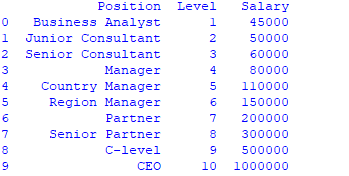Step 3 : Select all rows and column 1 from dataset to x and all rows and column 2 as y

 `x = data.iloc[:, 1:2].values  ` `print(x) ` `y = data.iloc[:, 2].values   `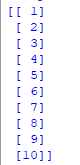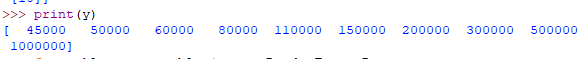Step 4 : Fit Random forest regressor to the dataset

 `# Fitting Random Forest Regression to the dataset ` `# import the regressor ` `from` `sklearn.ensemble ``import` `RandomForestRegressor ` ` `  ` ``# create regressor object ` `regressor ``=` `RandomForestRegressor(n_estimators ``=` `100``, random_state ``=` `0``) ` ` `  `# fit the regressor with x and y data ` `regressor.fit(x, y)   `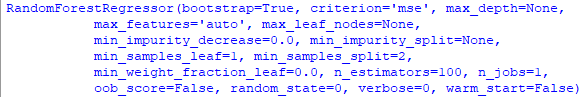Step 5 : Predicting a new result

 `Y_pred ``=` `regressor.predict(np.array([``6.5``]).reshape(``1``, ``1``))  ``# test the output by changing values `

Step 6 : Visualising the result

 `# Visualising the Random Forest Regression results ` ` `  `# arange for creating a range of values ` `# from min value of x to max  ` `# value of x with a difference of 0.01  ` `# between two consecutive values ` `X_grid ``=` `np.arange(``min``(x), ``max``(x), ``0.01``)  ` ` `  `# reshape for reshaping the data into a len(X_grid)*1 array,  ` `# i.e. to make a column out of the X_grid value                   ` `X_grid ``=` `X_grid.reshape((``len``(X_grid), ``1``)) ` ` `  `# Scatter plot for original data ` `plt.scatter(x, y, color ``=` `'blue'``)   ` ` `  `# plot predicted data ` `plt.plot(X_grid, regressor.predict(X_grid),  ` `         ``color ``=` `'green'``)  ` `plt.title(``'Random Forest Regression'``) ` `plt.xlabel(``'Position level'``) ` `plt.ylabel(``'Salary'``) ` `plt.show()`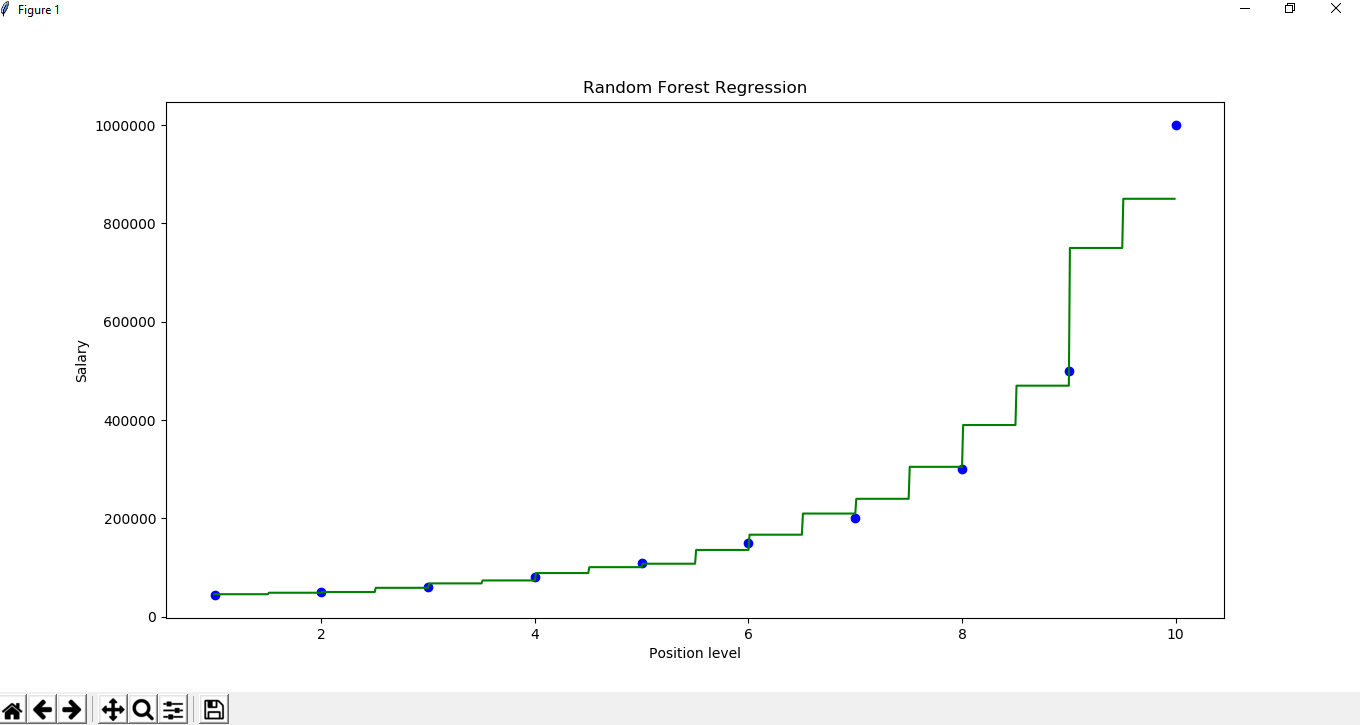My Personal Notes arrow_drop_upCheck out this Author's contributed articles.

If you like GeeksforGeeks and would like to contribute, you can also write an article using contribute.geeksforgeeks.org or mail your article to contribute@geeksforgeeks.org. See your article appearing on the GeeksforGeeks main page and help other Geeks.

Please Improve this article if you find anything incorrect by clicking on the "Improve Article" button below.

Article Tags :
Practice Tags :

2

Please write to us at contribute@geeksforgeeks.org to report any issue with the above content.